### Lecture Preview: Graph Implementation

(Suggested book reading: Programming Abstractions in C++, Chapter 18 sections 18.2 - 18.3, 18.5)

Today we will learn about how a graph class is actually implemented. The Stanford C++ library includes a class `BasicGraph` that contains several useful members related to graphs, vertices, edges, and paths. For example, if you wanted to create an object to represent the following graph: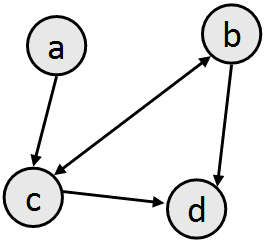Then you could create a `BasicGraph` as follows:

```BasicGraph graph;
```

But how exactly is `BasicGraph` implemented? What's inside of a `BasicGraph` object? How does it represent the vertices, edges, and so on? The answer is that there are multiple ways a graph can be implemented internally. Consider the following graph: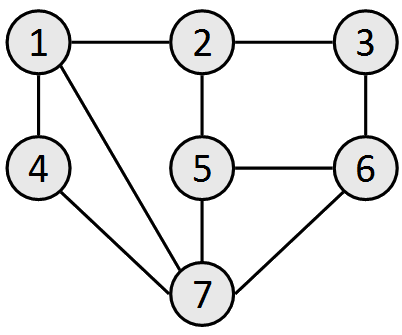One way to implement a graph is using an edge list, where the only structure you store is a list (vector) of the edges. The information about the vertices is implicit inside the edges. Here is an edge list for the above graph: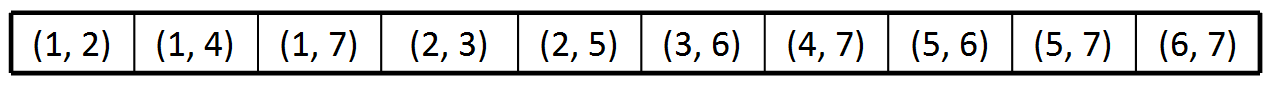A second way to implement a graph is using an adjacency list, where the only structure you store is a list of the vertices, where each vertex contains a nested list of other vertices that are its neighbors. The information about the edges is implicit inside the vertices' neighbor lists. Here is an adjacency list for the above graph: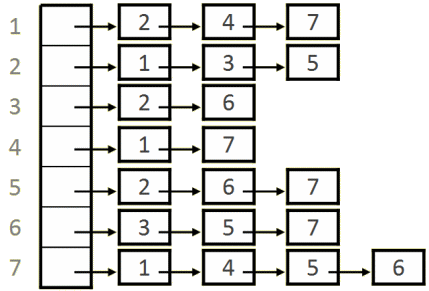A third way to implement a graph is using an adjacency matrix, where the only structure you store is a two-dimensional grid where each row represents a start vertex and each column represents an end vertex. The grid cell [i, j] stores information about the edge, if any, from vertex i to vertex j. Here is an adjacency matrix for the above graph: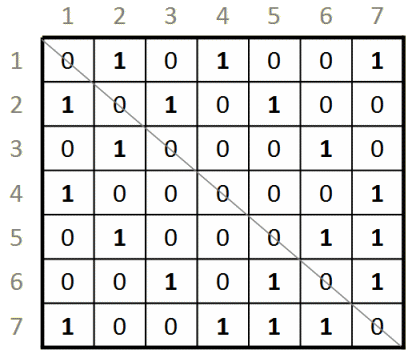We will discuss details of each approach as well as their relative pros/cons in lecture.

This document and its content are copyright © Marty Stepp, 2017. All rights reserved. Any redistribution, reproduction, transmission, or storage of part or all of the contents in any form is prohibited without the authors' expressed written permission.